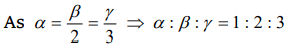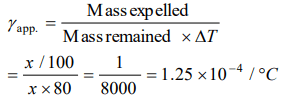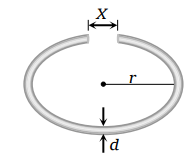## Thermal Properties of Matter Questions and Answers Part-6

1. Ratio among linear expansion coefficient $\left(\alpha\right)$, areal expansion coefficient $\left(\beta\right)$ and volume expansion coefficient $\left(\gamma\right)$ is
a) 1 : 2 : 3
b) 3 : 2 : 1
c) 4 : 3 : 2
d) None of these

Explanation:2. If on heating liquid through 80°C, the mass expelled is $\left(1/100\right)^{th}$  of mass still remaining, the coefficient of apparent expansion of liquid is
a) $1.25\times10^{-4}\diagup ^{\circ} C$
b) $12.5\times10^{-4}\diagup ^{\circ} C$
c) $1.25\times10^{-5}\diagup ^{\circ} C$
d) None of these

Explanation:3. In cold countries, water pipes sometimes burst, because
a) Pipe contracts
b) Water expands on freezing
c) When water freezes, pressure increases
d) When water freezes, it takes heat from pipes

Explanation: In anomalous expansion, water contracts on heating and expands on cooling in the range 0°C to 4°C. Therefore water pipes sometimes burst, in cold countries

4. A cylindrical metal rod of length L0 is shaped into a ring with a small gap as shown. On heating the systema) x decreases, r and d increase
b) x and r increase, d decreases
c) x, r and d all increase
d) Data insufficient to arrive at a conclusion

Explanation: On heating the system; x, r, d all increases, since the expansion of isotropic solids is similar to true photographic enlargement.

5. The length of a metallic rod is 5m at 0°C and becomes 5.01 m, on heating upto 100°C. The linear expansion of the metal will be
a) $2.33\times10^{-5}\diagup ^{\circ} C$
b) $6.0\times10^{-5}\diagup ^{\circ} C$
c) $4.0\times10^{-5}\diagup ^{\circ} C$
d) $2.0\times10^{-5}\diagup ^{\circ} C$

Explanation:6. A metal rod of silver at 0°C is heated to 100°C. It's length is increased by 0.19 cm. Coefficient of cubical expansion of the silver rod is
a) $5.7\times10^{-5}\diagup ^{\circ} C$
b) $0.63\times10^{-5}\diagup ^{\circ} C$
c) $1.9\times10^{-5}\diagup ^{\circ} C$
d) $16.1\times10^{-5}\diagup ^{\circ} C$

Explanation:7. A brass disc fits simply in a hole of a steel plate. The disc from the hole can be loosened if the system
a) First heated then cooled
b) First cooled then heated
c) Is heated
d) Is cooled

Explanation: Since, the coefficient of linear expansion of brass is greater than that of steel. On cooling, the brass contracts more, so, it get loosened.

8. An iron bar of length 10 m is heated from 0°C to 100°C. If the coefficient of linear thermal expansion of iron is $10\times10^{-6}\diagup ^{\circ} C$    , the increase in the length of bar is
a) 0.5 cm
b) 1.0 cm
c) 1.5 cm
d) 2.0 cm

Explanation:9. If a cylinder of diameter 1.0 cm at 30°C is to be solid into a hole of diameter 0.9997 cm in a steel plate at the same temperature, then minimum required rise in the temperature of the plate is : ( Coefficient of linear expansion of steel = $12\times10^{-6}\diagup ^{\circ} C$    )
a) 25°C
b) 35°C
c) 45°C
d) 55°C